x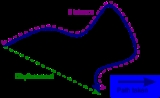Displacement (vector)Encyclopedia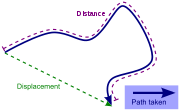A displacement is the shortest distance
Distance
Distance is a numerical description of how far apart objects are. In physics or everyday discussion, distance may refer to a physical length, or an estimation based on other criteria . In mathematics, a distance function or metric is a generalization of the concept of physical distance...

from the initial to the final position of a point P. Thus, it is the length of an imaginary straight path, typically distinct from the path actually travelled by P. A displacement vector represents the length and direction of that imaginary straight path.

A position vector expresses the position of a point P in space in terms of a displacement from an arbitrary reference point O (typically the origin of a coordinate system). Namely, it indicates both the distance and direction of an imaginary motion along a straight line from the reference position to the actual position of the point.

A displacement may be also described as a relative position: the final position of a point (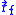) relative to its initial position (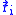), and a displacement vector can be mathematically defined as the difference between the final and initial position vectors: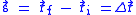In considering motions of objects over time the instantaneous velocity
Velocity
In physics, velocity is speed in a given direction. Speed describes only how fast an object is moving, whereas velocity gives both the speed and direction of the object's motion. To have a constant velocity, an object must have a constant speed and motion in a constant direction. Constant ...

of the object is the rate of change of the displacement as a function of time. The velocity then is distinct from the instantaneous speed
Speed
In kinematics, the speed of an object is the magnitude of its velocity ; it is thus a scalar quantity. The average speed of an object in an interval of time is the distance traveled by the object divided by the duration of the interval; the instantaneous speed is the limit of the average speed as...

which is the time rate of change of the distance traveled along a specific path.
The velocity may be equivalently defined as the time rate of change of the position vector. If one considers a moving initial position, or equivalenty a moving origin (e.g. an initial position or origin which is fixed to a train wagon, which in turn moves with respect to its rail track), the velocity of P (e.g. a point representing the position of a passenger walking on the train) may be referred to as a relative velocity, as opposed to an absolute velocity, which is computed with respect to a point which is considered to be "fixed in space" (such as, for instance, a point fixed on the floor of the train station).

For motion over a given interval of time, the displacement divided by the length of the time interval defines the average velocity
Velocity
In physics, velocity is speed in a given direction. Speed describes only how fast an object is moving, whereas velocity gives both the speed and direction of the object's motion. To have a constant velocity, an object must have a constant speed and motion in a constant direction. Constant ...

.

## Rigid body

In dealing with the motion of a rigid body
Rigid body
In physics, a rigid body is an idealization of a solid body of finite size in which deformation is neglected. In other words, the distance between any two given points of a rigid body remains constant in time regardless of external forces exerted on it...

, the term displacement may also include the rotation
Rotation
A rotation is a circular movement of an object around a center of rotation. A three-dimensional object rotates always around an imaginary line called a rotation axis. If the axis is within the body, and passes through its center of mass the body is said to rotate upon itself, or spin. A rotation...

s of the body. In this case, the displacement of a particle of the body is called linear displacement (displacement along a line), while the rotation is called angular displacement
Angular displacement
Angular displacement of a body is the angle in radians through which a point or line has been rotated in a specified sense about a specified axis....

.

## Derivatives

Velocity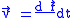Acceleration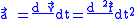Jolt/Jerk/Surge/Lurch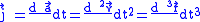Snap/Jounce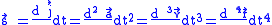Crackle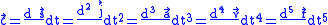Pop/Pounce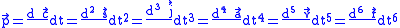Lock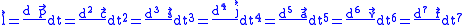Drop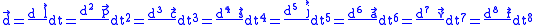Where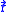is the position vector,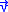is the velocity vector,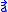is the acceleration vector,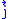is the jerk vector,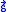is the snap vector,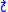is the crackle vector,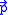is the pop vector,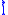is the lock vector, and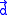is the drop vector.